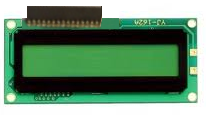﻿ HD44780 LCD Pinout

# HD44780 LCD PinoutThe HD44780 is a common and popular LCD screen where standard ASCII characters can be output to.

### Pinout

The HD44780 has 16 pinout terminals, as shown below:This is what each of the 16 pins stand for:

1. Ground- This is the ground of the LCD.
2. VCC- This is the pin where you place DC voltage so that the LCD can be powered. The voltage that the LCD receives is +3.3 to +5V.
4. Register Select Pin(RS)- If RS=0, this is Command Mode. If RS=1, this is Data Mode
5. Read/Write Pin- If R/W=0, this is Write Mode. If R/W=1, this is Read Mode.
6. Clock (Enable) Pin. The HD44780 is falling edge triggered.
7. Bit 0 (Not used in 4-bit operation)
8. Bit 1 (Not used in 4-bit operation)
9. Bit 2 (Not used in 4-bit operation)
10. Bit 3 (Not used in 4-bit operation)
11. Bit 4
12. Bit 5
13. Bit 6
14. Bit 7
15. Backlight Anode (+)- This is where you place the positive DC voltage if you want the backlight to be powered. The nominal operating voltage for LED backlights is 5V at full brightness. Connecting a potentiometer to this can dim the lights to the adjusted level that you want it. If the LCD has non-LED backlights, they often require higher voltages.
16. Backlight Cathode- This is where you connect the ground of the DC voltage for the LCD backlights.(-)